# R S Aggarwal Solutions for Class 10 Maths Chapter 16 Area of Circle, Sector and Segment Exercise 16B

R S Aggarwal Solutions for Class 10 Maths Chapter 16 exercise 16B of Area of Circle, Sector and Segment is available here. This exercise includes concepts such as Areas of Combination of plane figures and real life problems. Explore the various real life problems by solving this exercise. Find more learning resources on R S Aggarwal Solutions of Class 10 Chapter 16 to aid your exam preparation and streamline your last-minute revisions.

## Download PDF of R S Aggarwal Solutions for Class 10 Maths Chapter 16 Area of Circle, Sector and Segment Exercise 16B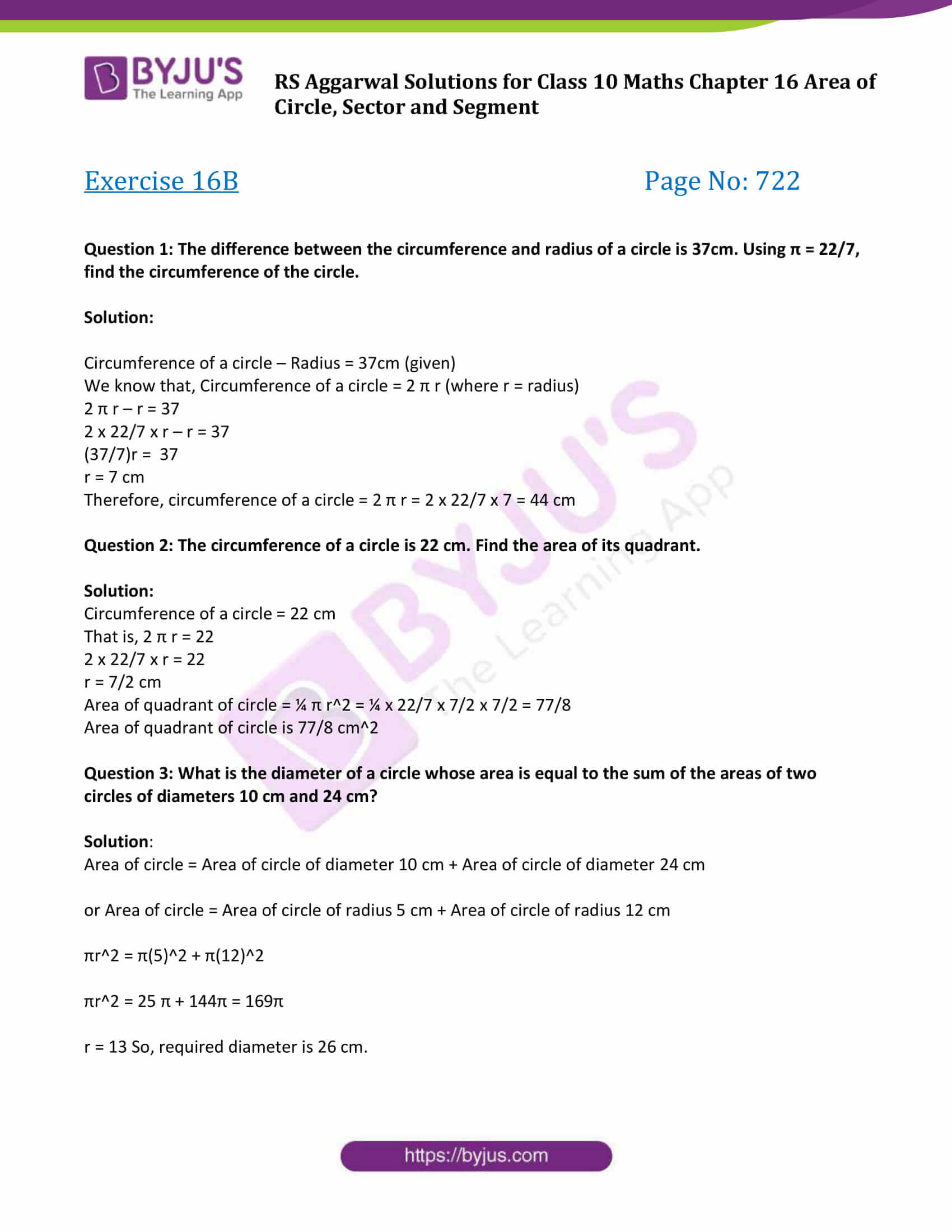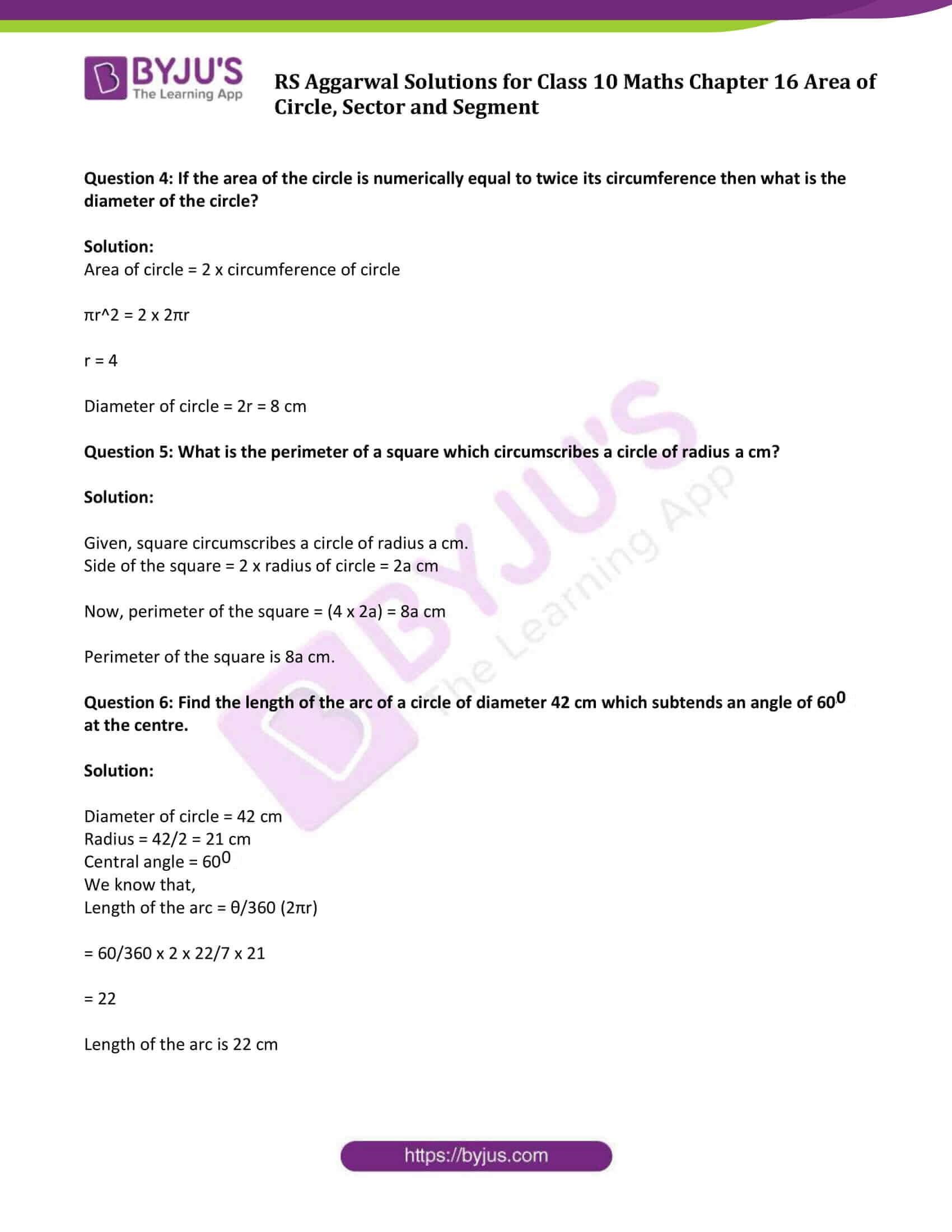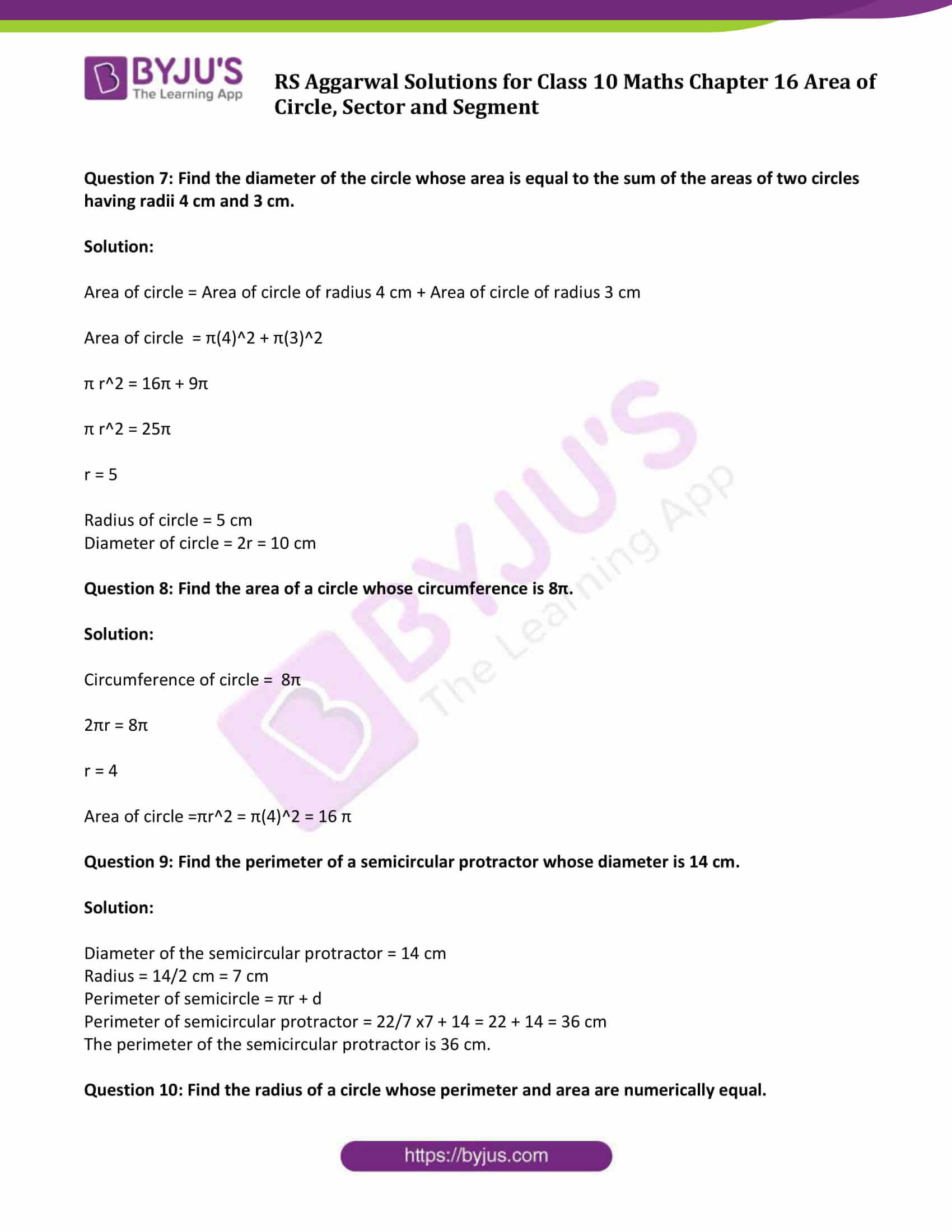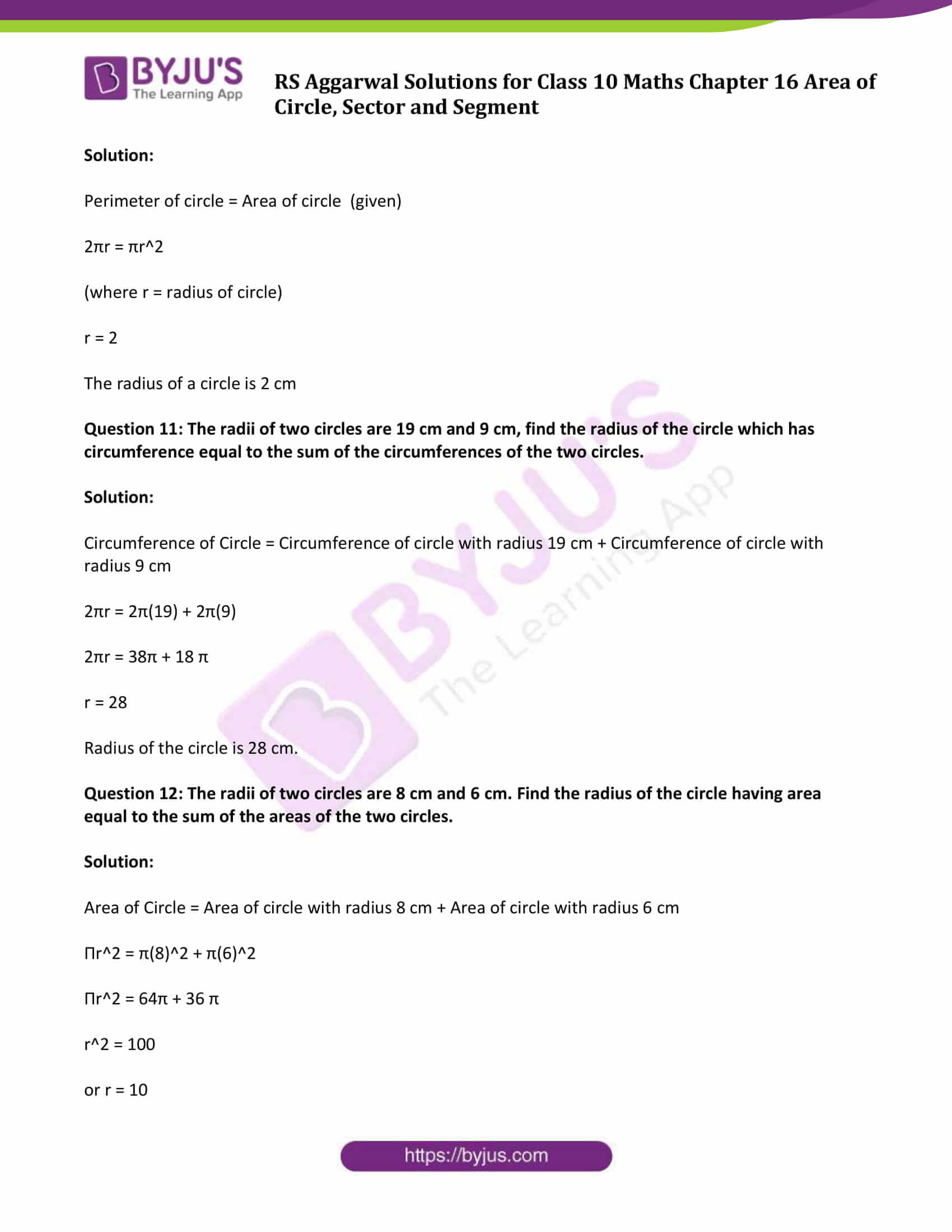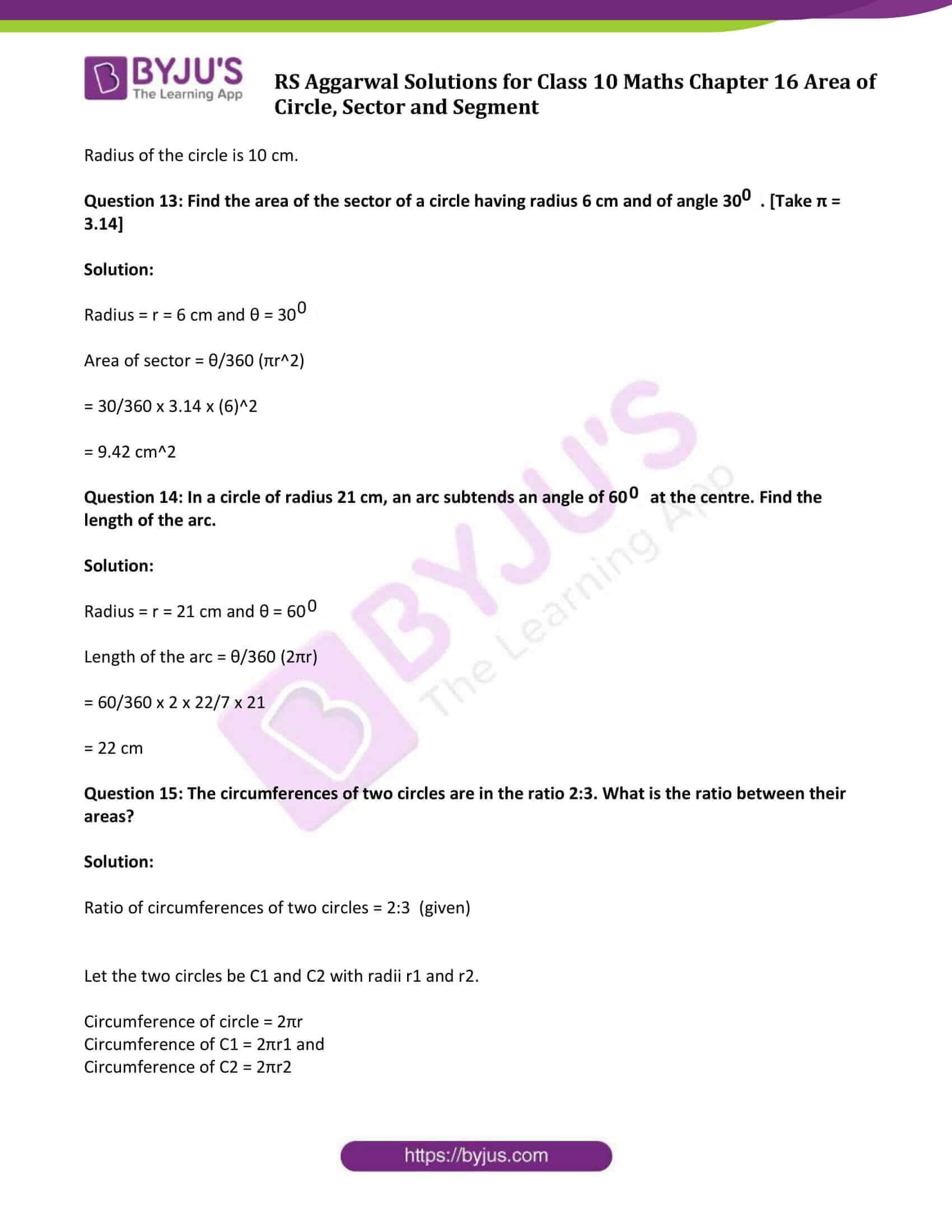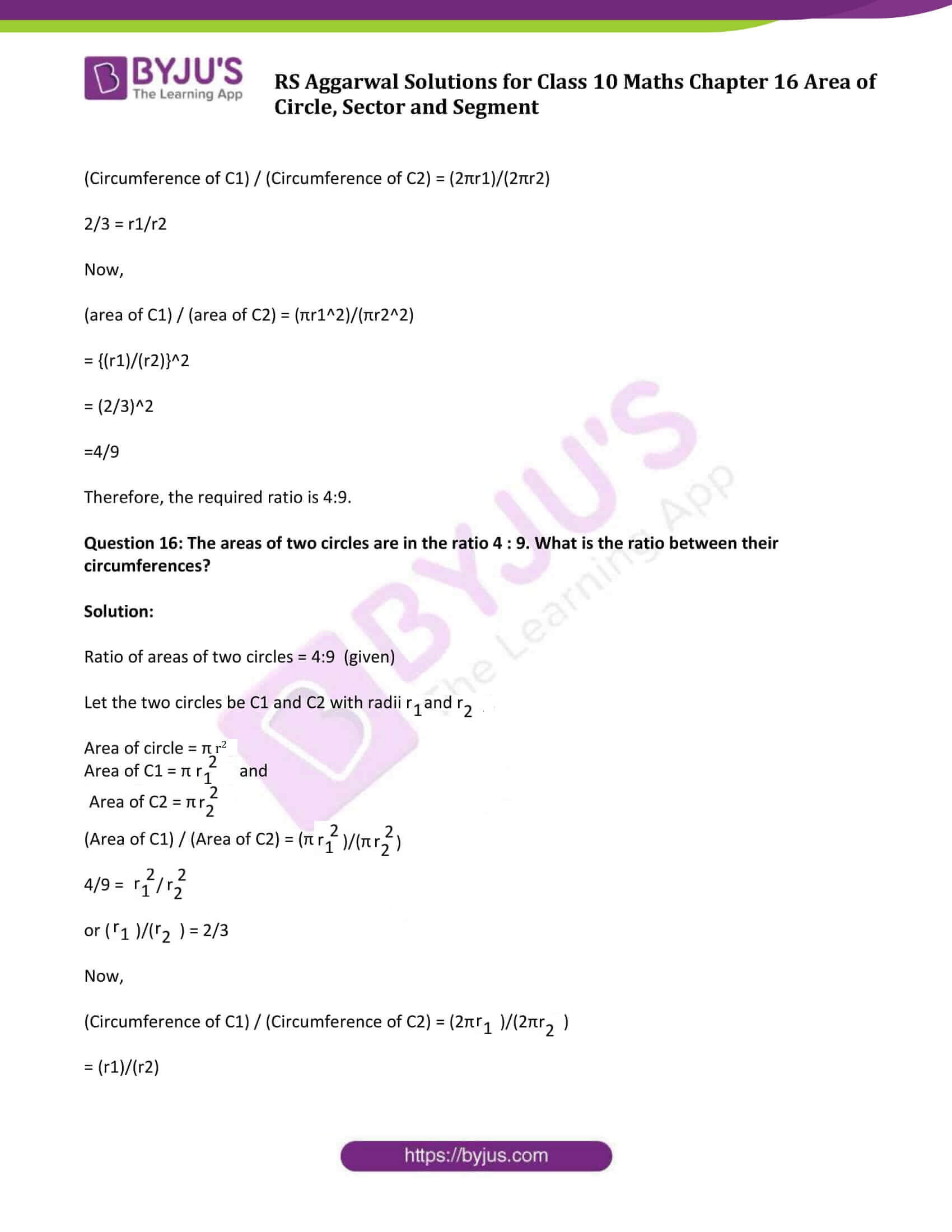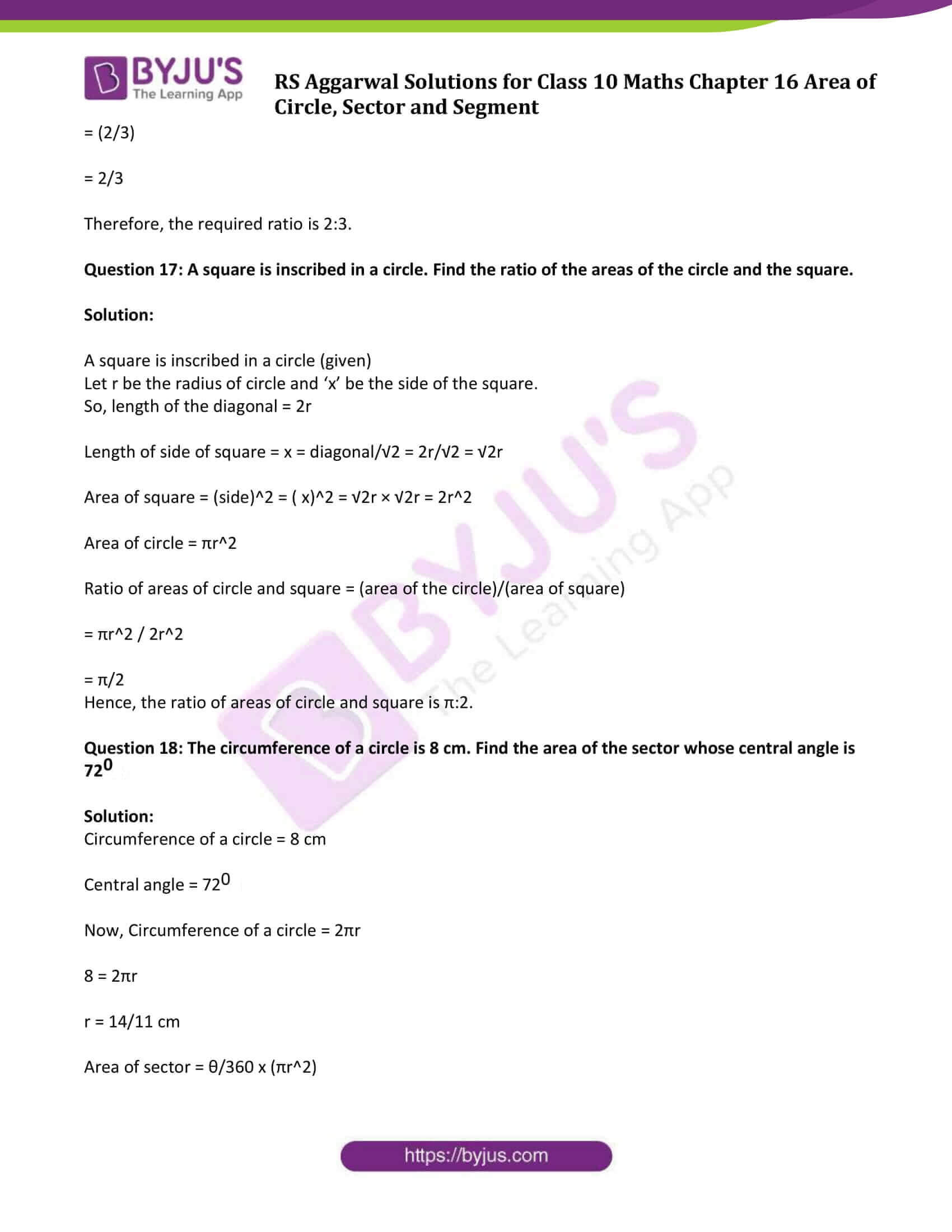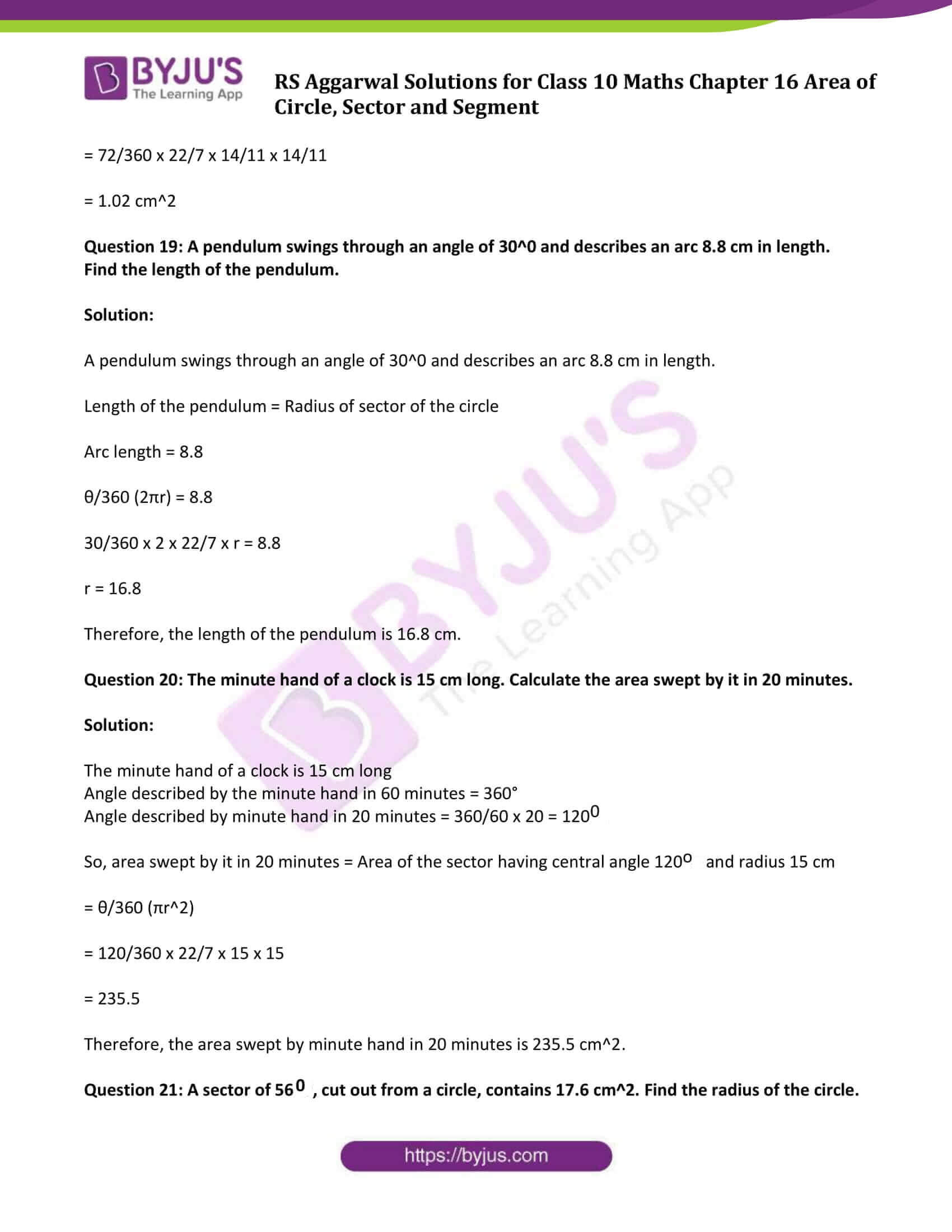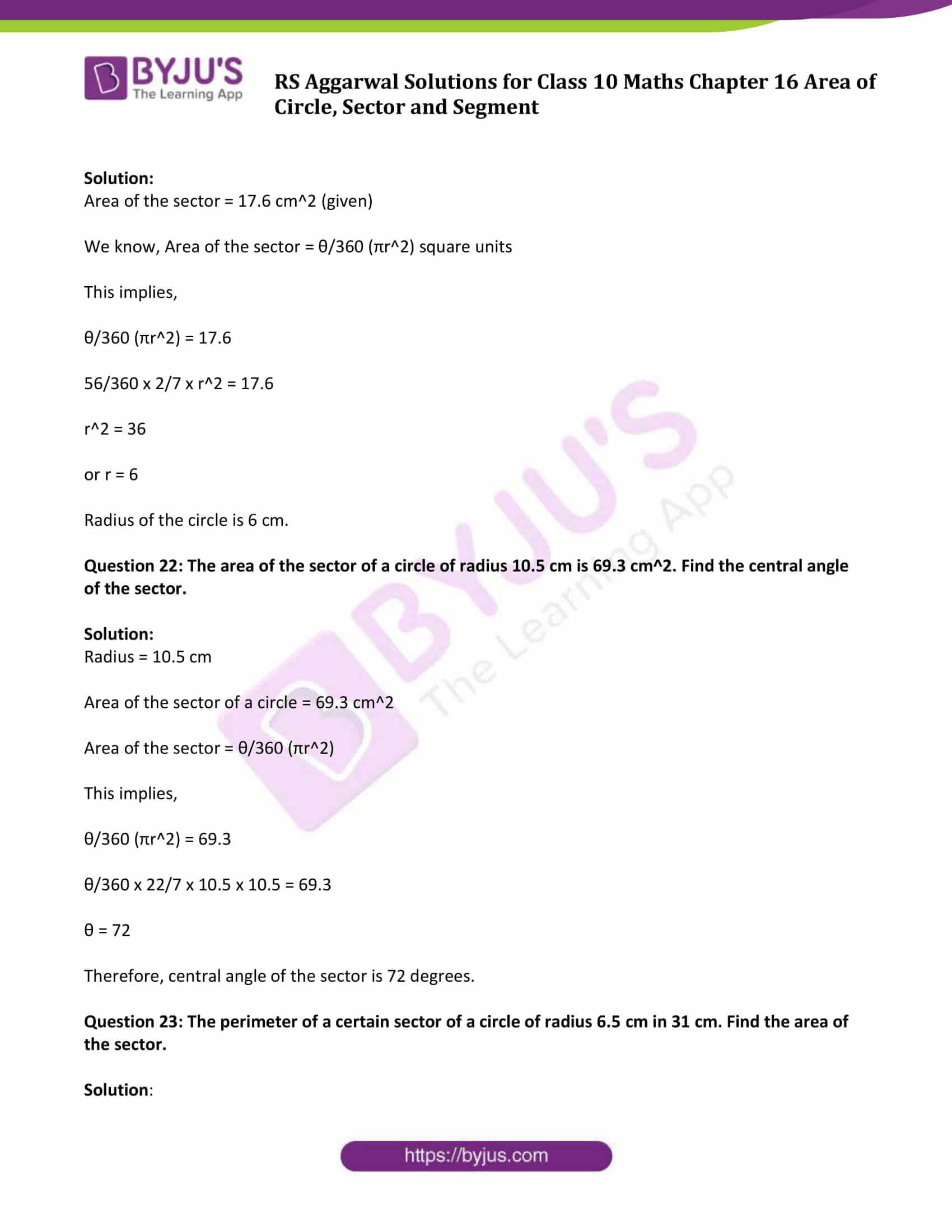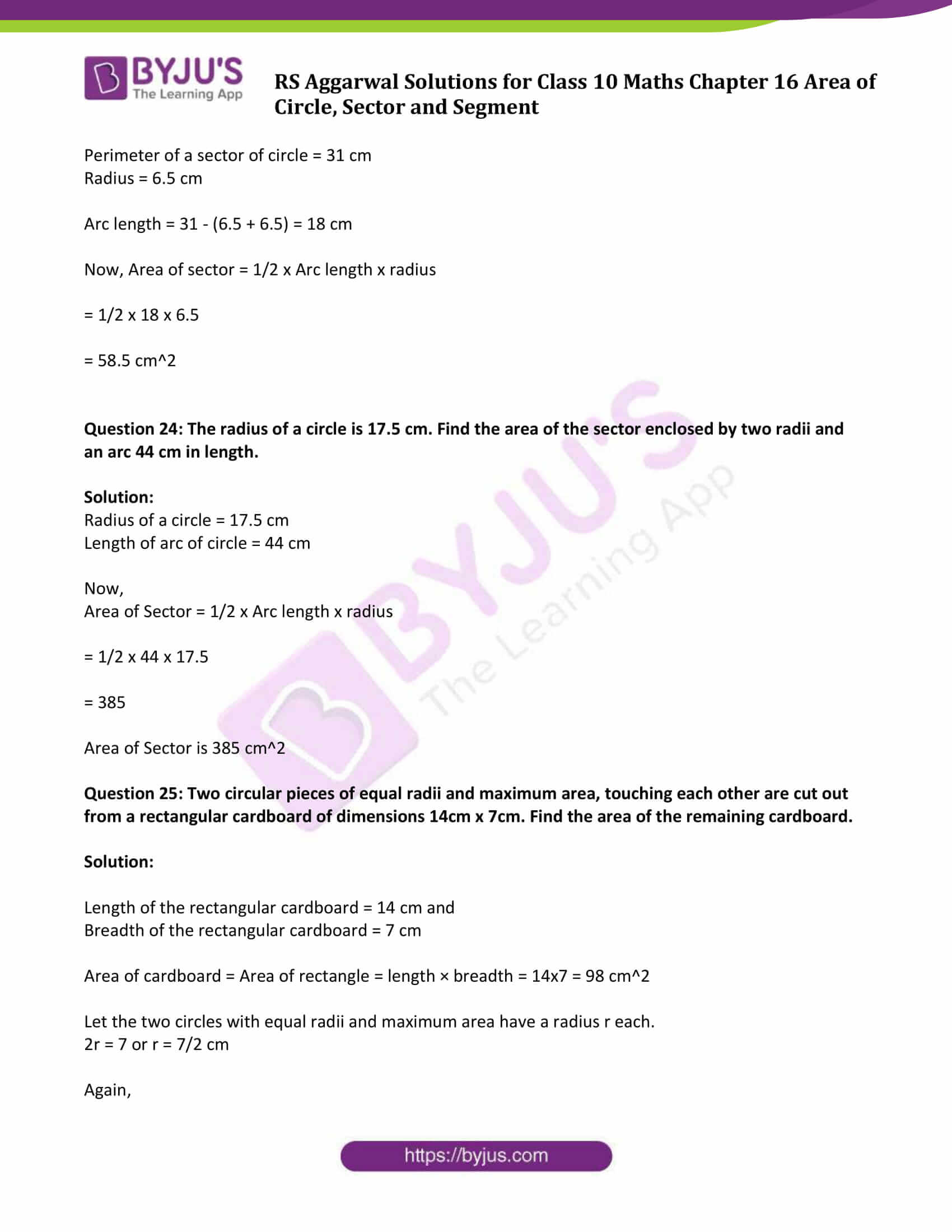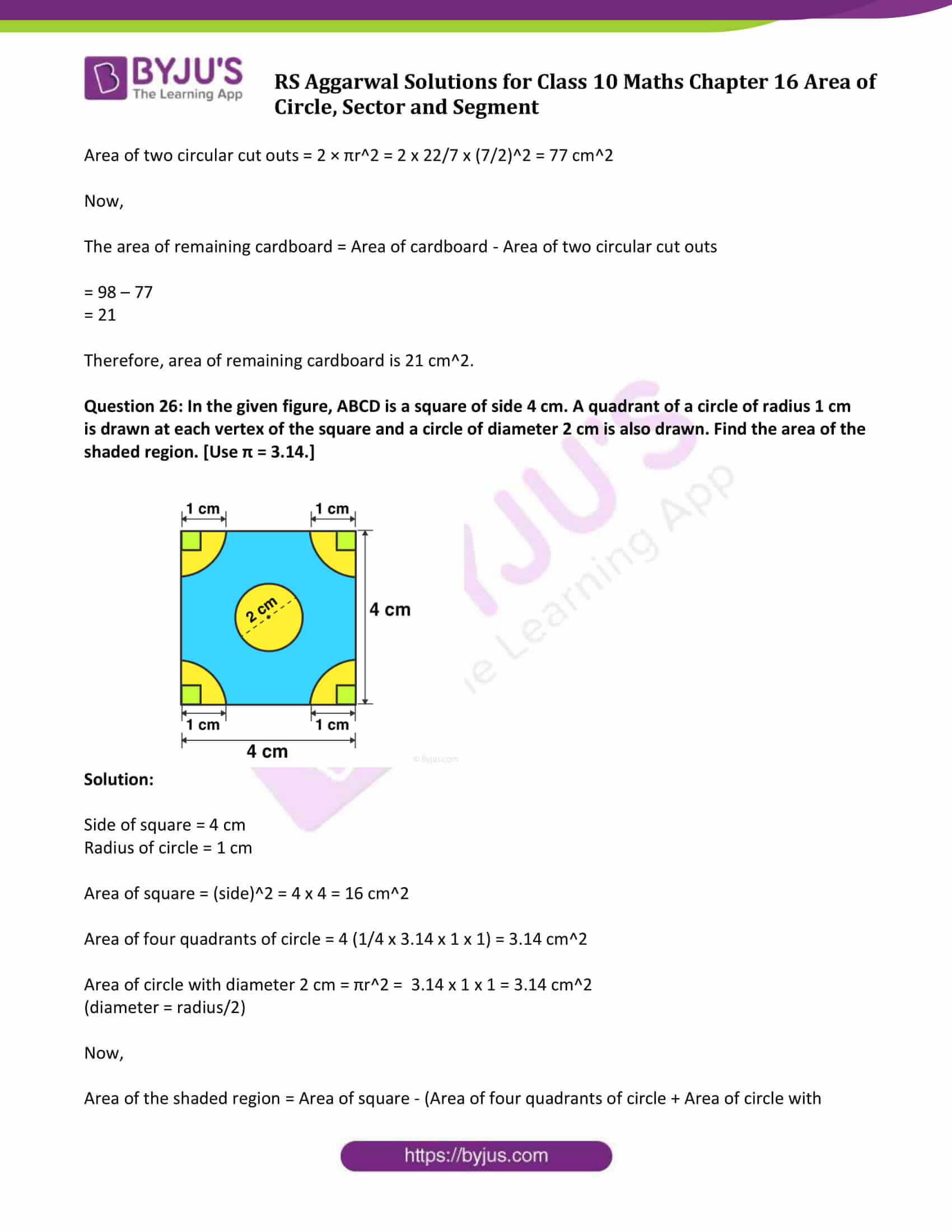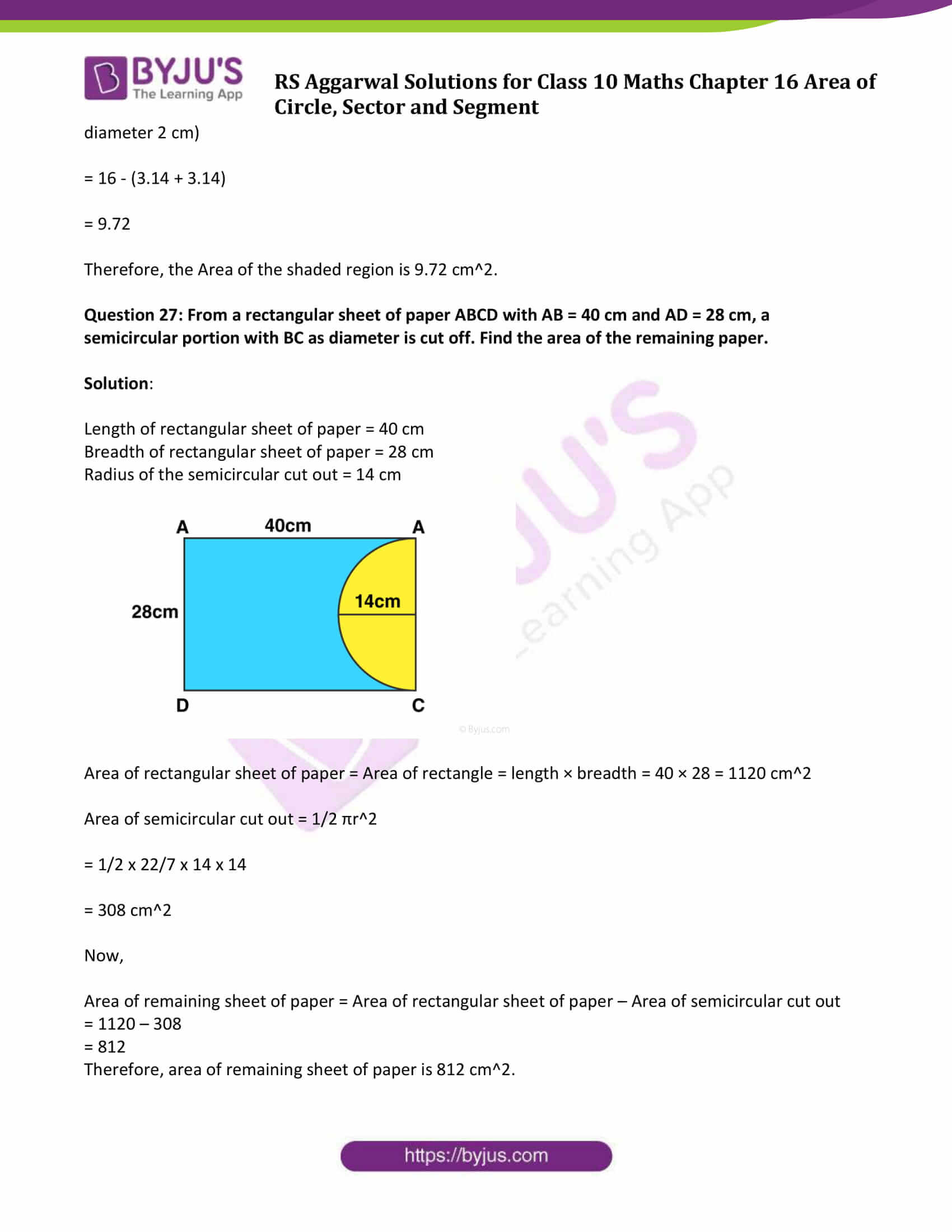### Access other exercise solutions of Class 10 Maths Chapter 16 Area of Circle, Sector and Segment

Exercise 16A Solutions: 30 Questions (Short Answers)

## Exercise 16B Page No: 722

Question 1: The difference between the circumference and radius of a circle is 37cm. Using π = 22/7, find the circumference of the circle.

Solution:

Circumference of a circle – Radius = 37cm (given)

We know that, Circumference of a circle = 2 π r (where r = radius)

2 π r – r = 37

2 x 22/7 x r – r = 37

(37/7)r = 37

r = 7 cm

Therefore, circumference of a circle = 2 π r = 2 x 22/7 x 7 = 44 cm

Question 2: The circumference of a circle is 22 cm. Find the area of its quadrant.

Solution:

Circumference of a circle = 22 cm

That is, 2 π r = 22

2 x 22/7 x r = 22

r = 7/2 cm

Area of quadrant of circle = ¼ π r2 = ¼ x 22/7 x 7/2 x 7/2 = 77/8

Area of quadrant of circle is 77/8 cm2

Question 3: What is the diameter of a circle whose area is equal to the sum of the areas of two circles of diameters 10 cm and 24 cm?

Solution:

Area of circle = Area of circle of diameter 10 cm + Area of circle of diameter 24 cm

or Area of circle = Area of circle of radius 5 cm + Area of circle of radius 12 cm

πr2 = π(5)2 + π(12)2

πr2 = 25 π + 144π = 169π

r = 13 So, required diameter is 26 cm.

Question 4: If the area of the circle is numerically equal to twice its circumference then what is the diameter of the circle?

Solution:

Area of circle = 2 x circumference of circle

πr2 = 2 x 2πr

r = 4

Diameter of circle = 2r = 8 cm

Question 5: What is the perimeter of a square which circumscribes a circle of radius a cm?

Solution:

Given, square circumscribes a circle of radius a cm.

Side of the square = 2 x radius of circle = 2a cm

Now, perimeter of the square = (4 x 2a) = 8a cm

Perimeter of the square is 8a cm.

Question 6: Find the length of the arc of a circle of diameter 42 cm which subtends an angle of 600 at the centre.

Solution:

Diameter of circle = 42 cm

Radius = 42/2 = 21 cm

Central angle = 600

We know that,

Length of the arc = θ/360 (2πr)

= 60/360 x 2 x 22/7 x 21

= 22

Length of the arc is 22 cm

Question 7: Find the diameter of the circle whose area is equal to the sum of the areas of two circles having radii 4 cm and 3 cm.

Solution:

Area of circle = Area of circle of radius 4 cm + Area of circle of radius 3 cm

Area of circle = π(4)2 + π(3)2

π r2 = 16π + 9π

π r2 = 25π

r = 5

Radius of circle = 5 cm

Diameter of circle = 2r = 10 cm

Question 8: Find the area of a circle whose circumference is 8π.

Solution:

Circumference of circle = 8π

2πr = 8π

r = 4

Area of circle =πr2 = π(4)2 = 16 π

Question 9: Find the perimeter of a semicircular protractor whose diameter is 14 cm.

Solution:

Diameter of the semicircular protractor = 14 cm

Radius = 14/2 cm = 7 cm

Perimeter of semicircle = πr + d

Perimeter of semicircular protractor = 22/7 x7 + 14 = 22 + 14 = 36 cm

The perimeter of the semicircular protractor is 36 cm.

Question 10: Find the radius of a circle whose perimeter and area are numerically equal.

Solution:

Perimeter of circle = Area of circle (given)

2πr = πr2

(where r = radius of circle)

r = 2

The radius of a circle is 2 cm

Question 11: The radii of two circles are 19 cm and 9 cm, find the radius of the circle which has circumference equal to the sum of the circumferences of the two circles.

Solution:

Circumference of Circle = Circumference of circle with radius 19 cm + Circumference of circle with radius 9 cm

2πr = 2π(19) + 2π(9)

2πr = 38π + 18 π

r = 28

Radius of the circle is 28 cm.

Question 12: The radii of two circles are 8 cm and 6 cm. Find the radius of the circle having area equal to the sum of the areas of the two circles.

Solution:

Area of Circle = Area of circle with radius 8 cm + Area of circle with radius 6 cm

Πr2 = π(8)2 + π(6)2

Πr2 = 64π + 36 π

r2 = 100

or r = 10

Radius of the circle is 10 cm.

Question 13: Find the area of the sector of a circle having radius 6 cm and of angle 300. [Take π = 3.14]

Solution:

Radius = r = 6 cm and θ = 300

Area of sector = θ/360 (πr2)

= 30/360 x 3.14 x (6)2

= 9.42 cm2

Question 14: In a circle of radius 21 cm, an arc subtends an angle of 600 at the centre. Find the length of the arc.

Solution:

Radius = r = 21 cm and θ = 600

Length of the arc = θ/360 (2πr)

= 60/360 x 2 x 22/7 x 21

= 22 cm

Question 15: The circumferences of two circles are in the ratio 2:3. What is the ratio between their areas?

Solution:

Ratio of circumferences of two circles = 2:3 (given)

Let the two circles be C1 and C2 with radii r1 and r2.

Circumference of circle = 2πr

Circumference of C1 = 2πr1 and

Circumference of C2 = 2πr2

(Circumference of C1) / (Circumference of C2) = (2πr1)/(2πr2)

2/3 = r1/r2

Now,

(area of C1) / (area of C2) = (πr12)/(πr22)

= {(r1)/(r2)}2

= (2/3)2

=4/9

Therefore, the required ratio is 4:9.

Question 16: The areas of two circles are in the ratio 4 : 9. What is the ratio between their circumferences?

Solution:

Ratio of areas of two circles = 4:9 (given)

Let the two circles be C1 and C2 with radii r1 and r2.

Area of circle = πr2

Area of C1 = πr12 and

Area of C2 = πr22

(Area of C1) / (Area of C2) = (πr12)/(πr22)

4/9 = r12/r22

or (r1)/(r2) = 2/3

Now,

(Circumference of C1) / (Circumference of C2) = (2πr1)/(2πr2)

= (r1)/(r2)

= (2/3)

= 2/3

Therefore, the required ratio is 2:3.

Question 17: A square is inscribed in a circle. Find the ratio of the areas of the circle and the square.

Solution:

A square is inscribed in a circle (given)

Let r be the radius of circle and ‘x’ be the side of the square.

So, length of the diagonal = 2r

Length of side of square = x = diagonal/√2 = 2r/√2 = √2r

Area of square = (side)2 = ( x)2 = √2r × √2r = 2r2

Area of circle = πr2

Ratio of areas of circle and square = (area of the circle)/(area of square)

= πr2 / 2r2

= π/2

Hence, the ratio of areas of circle and square is π:2.

Question 18: The circumference of a circle is 8 cm. Find the area of the sector whose central angle is 720.

Solution:

Circumference of a circle = 8 cm

Central angle = 720

Now, Circumference of a circle = 2πr

8 = 2πr

r = 14/11 cm

Area of sector = θ/360 x (πr2)

= 72/360 x 22/7 x 14/11 x 14/11

= 1.02 cm2

Question 19: A pendulum swings through an angle of 30^0 and describes an arc 8.8 cm in length. Find the length of the pendulum.

Solution:

A pendulum swings through an angle of 30^0 and describes an arc 8.8 cm in length.

Length of the pendulum = Radius of sector of the circle

Arc length = 8.8

θ/360 (2πr) = 8.8

30/360 x 2 x 22/7 x r = 8.8

r = 16.8

Therefore, the length of the pendulum is 16.8 cm.

Question 20: The minute hand of a clock is 15 cm long. Calculate the area swept by it in 20 minutes.

Solution:

The minute hand of a clock is 15 cm long

Angle described by the minute hand in 60 minutes = 360°

Angle described by minute hand in 20 minutes = 360/60 x 20 = 1200

So, area swept by it in 20 minutes = Area of the sector having central angle 120o and radius 15 cm

= θ/360 (πr2)

= 120/360 x 22/7 x 15 x 15

= 235.5

Therefore, the area swept by minute hand in 20 minutes is 235.5 cm2.

Question 21: A sector of 560, cut out from a circle, contains 17.6 cm2. Find the radius of the circle.

Solution:

Area of the sector = 17.6 cm2 (given)

We know, Area of the sector = θ/360 (πr2) square units

This implies,

θ/360 (πr2) = 17.6

56/360 x 2/7 x r2 = 17.6

r2 = 36

or r = 6

Radius of the circle is 6 cm.

Question 22: The area of the sector of a circle of radius 10.5 cm is 69.3 cm2. Find the central angle of the sector.

Solution:

Area of the sector of a circle = 69.3 cm2

Area of the sector = θ/360 (πr2)

This implies,

θ/360 (πr2) = 69.3

θ/360 x 22/7 x 10.5 x 10.5 = 69.3

θ = 72

Therefore, central angle of the sector is 72 degrees.

Question 23: The perimeter of a certain sector of a circle of radius 6.5 cm in 31 cm. Find the area of the sector.

Solution:

Perimeter of a sector of circle = 31 cm

Arc length = 31 – (6.5 + 6.5) = 18 cm

Now, Area of sector = 1/2 x Arc length x radius

= 1/2 x 18 x 6.5

= 58.5 cm2

Question 24: The radius of a circle is 17.5 cm. Find the area of the sector enclosed by two radii and an arc 44 cm in length.

Solution:

Radius of a circle = 17.5 cm

Length of arc of circle = 44 cm

Now,

Area of Sector = 1/2 x Arc length x radius

= 1/2 x 44 x 17.5

= 385

Area of Sector is 385 cm2

Question 25: Two circular pieces of equal radii and maximum area, touching each other are cut out from a rectangular cardboard of dimensions 14cm x 7cm. Find the area of the remaining cardboard.

Solution:

Length of the rectangular cardboard = 14 cm and

Breadth of the rectangular cardboard = 7 cm

Area of cardboard = Area of rectangle = length × breadth = 14×7 = 98 cm2

Let the two circles with equal radii and maximum area have a radius r each.

2r = 7 or r = 7/2 cm

Again,

Area of two circular cut outs = 2 × πr2 = 2 x 22/7 x (7/2)2 = 77 cm2

Now,

The area of remaining cardboard = Area of cardboard – Area of two circular cut outs

= 98 – 77

= 21

Therefore, area of remaining cardboard is 21 cm2.

Question 26: In the given figure, ABCD is a square of side 4 cm. A quadrant of a circle of radius 1 cm is drawn at each vertex of the square and a circle of diameter 2 cm is also drawn. Find the area of the shaded region. [Use π = 3.14.]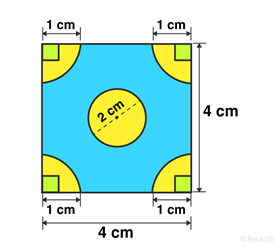Solution:

Side of square = 4 cm

Radius of circle = 1 cm

Area of square = (side)2 = 4 x 4 = 16 cm2

Area of four quadrants of circle = 4 (1/4 x 3.14 x 1 x 1) = 3.14 cm2

Area of circle with diameter 2 cm = πr2 = 3.14 x 1 x 1 = 3.14 cm2

Now,

Area of the shaded region = Area of square – (Area of four quadrants of circle + Area of circle with diameter 2 cm)

= 16 – (3.14 + 3.14)

= 9.72

Therefore, the Area of the shaded region is 9.72 cm2.

Question 27: From a rectangular sheet of paper ABCD with AB = 40 cm and AD = 28 cm, a semicircular portion with BC as diameter is cut off. Find the area of the remaining paper.

Solution:

Length of rectangular sheet of paper = 40 cm

Breadth of rectangular sheet of paper = 28 cm

Radius of the semicircular cut out = 14 cm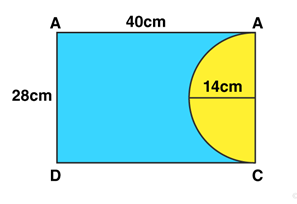Area of rectangular sheet of paper = Area of rectangle = length × breadth = 40 × 28 = 1120 cm2

Area of semicircular cut out = 1/2 πr2

= 1/2 x 22/7 x 14 x 14

= 308 cm2

Now,

Area of remaining sheet of paper = Area of rectangular sheet of paper – Area of semicircular cut out

= 1120 – 308

= 812

Therefore, area of remaining sheet of paper is 812 cm2.

Class 10 Maths Chapter 16 Area of Circle, Sector and Segment Exercise 16B is based on the topic, Areas of Combination of plane figures. In this exercise, students can explore how to solve problems such as, if the radii of two circles are given then find the radius of the circle having an area equal to the sum of the areas of the two circles.